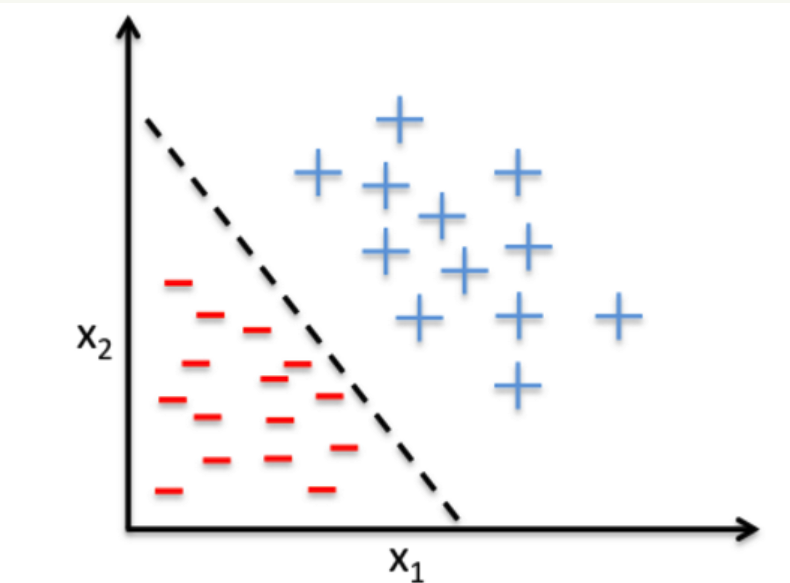# 感知机原理

## 感知机的原理及预备知识### 预备的数学知识

• 点到线的距离：直线方程为$Ax+By+C=0$,点P的坐标为$(x_0,y_0)$，那么点到直线的距离为：
$d = \frac{Ax_0+By_0 + C}{\sqrt{A^2 +B^2}}$
• 样本到超平面的距离： 假设超平面是$h=w \cdot x + b$,其中$w=(w_0, w_1,...,w_m), x=(x_0,x_1,...,x_m)$，样本点$x'$到超平面的距离为：
$d = \frac{w \cdot x' + b}{||w||}$
其中$||w||$是w的L2正则项，即$||w|| = \sqrt{w_0^2 + w_1^2 + ...+w_m^2}$
• 超平面：超平面是在空间$R^d$中的一个子空间$R^{d-1}$，在2维空间中的超平面就是一条直线，在3维空间中的超平面就是一个平面。

## 感知机模型

$(x^0_1,x^0_2,...,x^0_n,y_0), (x^1_1,x^1_2,...,x^1_n,y_1),...,(x^m_1,x^m_2,...,x^m_n,y_m)$

$w_0 + w_1x_1 + ... + w_nx_n = 0$

$sign(x) \begin{cases} -1 & x<0 \\ 1 & x\geq0 \end{cases}$

## 感知机的损失函数

$Loss(w,b) = - \frac{1}{||w||} \sum_{x_i \in M} y_i(w\cdot x_i +b)$

$Loss(w,b) = - \sum_{x_i \in M}y_i(w \cdot x_i +b)$

## 感知机学习算法

$Loss(w,b) = \mathop{\arg \min}\limits_{w,b} (- \sum_{x_i \in M}y_i(w\cdot x_i +b))$

### 原始形式的算法

1. 赋初值$w_0,b_0$
2. 选取数据点$(x_i, y_i)$
3. 判断该数据点是否为当前模型的误分类点，即如果$y_i(w\cdot x_i+b)<=0$，就更新：
$w = w + \eta y_ix_i$
$b = b + \eta y_i$
4. 转到2，直到训练集中没有误分类的点

### 对偶形式的算法

$w = \sum_{i=1}^{N}n_i\eta y_ix_i$
$b = \sum_{i=1}^{N} n_i \eta y_i$

$f(x) = sign(w\cdot x+b) = sign(\sum_{j=1}^{N}n_j\eta y_jx_j\cdot x + \sum_{j=1}^{N}n_j\eta y_j)$

1. 初始时$n_i=0,i=1,2...N$
2. 在训练集中选取数据点$(x_i, y_i)$
3. 如果$y_i(\sum_{j=1}^Nn_j\eta y_jx_j\cdot x_i + \sum_{j=1}^{N}n_j\eta y_j)\leq 0$，就可以进行更新：$n_i \leftarrow n_i +1$
4. 转到2直至没有误分类数据

$G=[x_i,x_j]_{N \times N}$

### 原始形式和对偶形式的选择

• 在向量维度(特征数)过高时，计算内积非常耗时，应该选择对偶形式算法加速
• 在向量个数(样本数)过多时，每次累计计算就比较耗时，应该选择原始算法

©️2019 CSDN 皮肤主题: 大白 设计师: CSDN官方博客